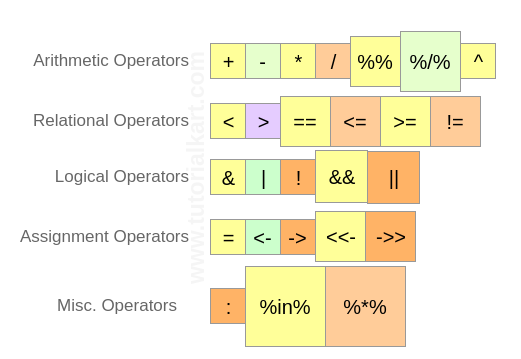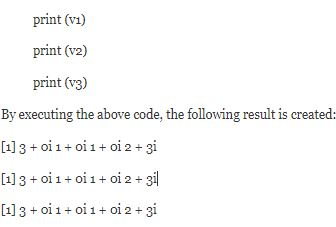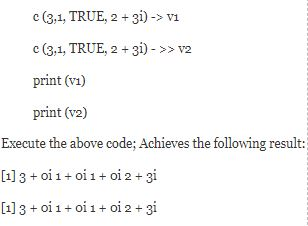# blog posts# An operator is a symbol that tells a compiler to perform certain mathematical or logical operations.

## Types of operators

In R programming, we have the following types of operators:

• Arithmetic operators
• Relational operators
• Logical operators
• Assignment operators
• Miscellaneous operators

### Arithmetic operators

The table below shows the arithmetic operators supported by the R programming language. Operators act as a vector on each element.

 operator Operator explanation Example + Combines two vectors. v <- c (2,5.5,6) t <- c (8, 3, 4) print (v + t) Execute the above code; Creates the following result:  10.0 8.5 10.0 – Subtracts the second vector from the first vector. v <- c (2,5.5,6) t <- c (8, 3, 4) print (vt) Execute the above code; Creates the following result:  -6.0 2.5 ۲ 2.0 * Multiplies two vectors v <- c (2,5.5,6) t <- c (8, 3, 4) print (v * t) Execute the above code; Creates the following result:  16.0 16.5 24.0 / Divides the first vector by the second vector v <- c (2,5.5,6) t <- c (8, 3, 4) print (v / t) Execute the above code; Creates the following result:  0. 250,000 1. 833333 1.500000 %% Returns the remainder of dividing the first vector by the second vector v <- c (2,5.5,6) t <- c (8, 3, 4) print (v %% t) Execute the above code; Creates the following result:  2.0 ٫ 2.5 ۲ 2.0 % /% Creates the result of dividing the first vector by the second (outside the part) v <- c (2,5.5,6) t <- c (8, 3, 4) print (v% /% t) Execute the above code; Creates the following result:  0 1 1 ^ Bring the first vector to the power of the second vector. v <- c (2,5.5,6) t <- c (8, 3, 4) print (v ^ t) Execute the above code; Creates the following result:  256,000 166,375 1296,000

### Relational operators

The table below shows the relational operators supported by the R programming language. Each element of the first vector is compared with the corresponding element of the second vector. The result is a comparison of a boolean variable.

 operator Explain its function Example > Checks whether each element of the first vector; Is it larger than the corresponding elements in the second vector or not? v <- c (2,5.5,6,9) t <- c (8,2.5,14,9) print (v> t) Execution of the above code achieves the following result:  FALSE TRUE FALSE FALSE < Checks whether each element of the first vector; Is it smaller than the corresponding element in the second vector or not? v <- c (2,5.5,6,9) t <- c (8,2.5,14,9) print (v = t) Execution of the above code achieves the following result:  TRUE FALSE TRUE TRUE ! = Checks whether each element of the first vector is equal to the corresponding element in the second vector. v <- c (2,5.5,6,9) t <- c (8,2.5,14,9) print (v! = t) Execution of the above code achieves the following result:  TRUE TRUE TRUE FALSE

### Logical operators

The following is a table showing the logical operators that are supported in the R programming language. These operators can only be applied to vectors that are of the logical or complex type. All numbers greater than 1 are considered as the logical value of TRUE.

Each element of the first vector; Is compared with the corresponding element in the second vector. The result is shown as a boolean value.

 Operator Description Example & It is called element-to-element logical AND operator. This operator combines each element of the first vector with the corresponding element in the second vector, and if both variables are TRUE; Returns TRUE output. v <- c (3,1, TRUE, 2 + 3i) t <- c (4.1, FALSE, 2 + 3i) print (v & t) This code; Achieves the following result:  TRUE TRUE FALSE TRUE | The logical operator OR is called element to element, and combines each element of the first vector with the corresponding element in the second vector, and if one of the variables is TRUE; Returns TRUE output v <- c (3.0, TRUE, 2 + 2i) t <- c (4.0, FALSE, 2 + 3i) print (v | t) Execution of this code will give the following result:  TRUE FALSE TRUE TRUE ! This operator is called the NOT logical operator; Takes each element of the vector and returns the opposite logical value. v <- c (3.0, TRUE, 2 + 2i) print (! v) Executing the above code returns the following result:  FALSE TRUE FALSE FALSE

The logical operator considers && and only the first element of the vectors and creates a vector at the output that has only one element.

 Operator Explain the function of the operator Example && It is called the logical AND operator. Take the first element of both vectors and only if both elements are TRUE; Returns the TRUE statement. v <- c (3.0, TRUE, 2 + 2i) t <- c (1,3, TRUE, 2 + 3i) print (v && t) Executing the above code produces the following result:  TRUE || The OR operator is called logical and takes the first element of both vectors and returns TRUE if one of them is TRUE. v <- c (0,0, TRUE, 2 + 2i) t <- c (0.3, TRUE, 2 + 3i) print (v || t) Executing the above code produces the following result:  FALSE

### Assignment operators

These operators are used to assign values ​​to variables.

 Operators Explain their function Example <- Or = Or << – It is called left assignment. v1 <- c (3,1, TRUE, 2 + 3i) v2 << – c (3,1, TRUE, 2 + 3i) v3 = c (3,1, TRUE, 2 + 3i)-> Or – >> Is called the right allocation.### Miscellaneous operators

These operators are used for specific purposes and are generally not mathematical or logical.

 Operator Explain the function of the operator Example : Two-point operator; Which creates a series of consecutive numbers for a vector. v <- 2: 8 print (v) Execute this code; It has the following result:  2 3 4 5 6 7 8 % in% This operator is used to determine whether an element belongs to a vector or not. v1 <- 8 v2 <- 12 t <- 1:10 print (v1% in% t) print (v2% in% t) Execute the above code; The following is the result:  TRUE  FALSE % *% This operator is used to multiply a matrix in its output. M = matrix (c (2,6,5,1,10,4), nrow = 2, ncol = 3, byrow = TRUE) t = M% *% t (M) print (t) Execution of the above code achieves the following result: [, 1] [, 2] [1,] 65 82 [2,] 82 117